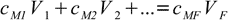# Concentration calculation

CASC concentration calculator

operating systems:
XP, Vista, 7, 8, 10

single user license price:
€49.95 - approximately \$65

Buy Now!

download 30-day free trial!
30-day money back guarantee!

## Concentration lectures » dilution and mixing

Calculations of final concentration of the substance during dilution and solution mixing are based on the mass balance of the solute - whatever you put into the solution stays there. Assuming cM and V are molar concentrations and volumes respectively of mixed solutions (indexed with digits) and the final solution volume and concentration are VF and CF respectively, we get the following formula:12.1

To reiterate: sum on the left represents total amount of substance in the combined solutions (in moles), expression on the right reflects the fact that final solution must contain exactly the same amount of substance.

In the case of a dilution this formula simplifies to12.2

While both formulas are correct they are sometimes of no use as they don't give a clue about how to calculate volume of the mixture. In the case of diluted solutions you may assume final volume is the sum of all volumes. However, due to the density changes and/or volume contractions the final volume doesn't have to equal sum of all the volumes mixed. Even a density tables are of no real help, as you can't calculate exact molarity of a solution without knowing its volume, you can't calculate a volume without knowing the density, and the density is a function of molarity that you can't calculate.

The easiest way to avoid such problems is to do the calculations using mass percentages and solution masses instead of volumes. In this case we will always refer to density tables knowing the concentration.

If the starting solution amounts are given as volumes, first step will be to convert them to masses using density tables. Then we will use the mass percentage definition12.3

and rather obvious equation12.4

to find substance and solvent masses for all solutions used:12.512.6

Next step is to add masses of substance from all solutions and masses of solvent from all solutions and to use definition 12.3 to calculate mass percentage. For this known mass percentage (and it is the correct value, not disturbed by any density/volume related problems) we can check density in tables and calculate volume of solution.

It is worth of noting here that mixture and dilution calculator built into our program CASC does all dilution/mixing calculations automatically, thanks to built in density tables.